# Grain size influence on the thermal properties of stabilized zirconia-nano spinel (Al2O3-MgO) composites

Document Type : Research Paper

Authors

1 Department of Physics, University of Kashan, Kashan, Iran

2 Instite of Nanoscience and Nanotechnology, University of Kashan, Kashan, Iran

3 Department of Applied Sciences, University of Technology, Baghdad, Iraq

Abstract

Abstract. Cubic crystalline oxides such as Y2O3 and MgO is used to stabalized zirconia phases at high temperature. In this paper the Y2O3 powder was added to stabilized zirconia and preparing a mixture of MgAl2O4 Spinel by mixing 1mol of nano-MgO with 1 mol of nano-Al2O3 powders. The spinel was added to the Y2O3-ZrO2 by various weight percentage (5, 10, 15, 20 and 25 wt%), and after that the specimens was prepared by axial pressing and sintered at 1550 oC for 4 hours as soaking time. The grain size was tested by using Atomic Force Microscopy (AFM) and calculated for surface of specimens and it was found to be decreasing (> 0.6 µm) to (~ 108 nm). The thermal properties were clearly influenced by the structural characteristics of the MAS. The thermal conductivity and thermal diffusion was decreased slightly from 5.7 to 5.5 W/m.K and from 2.5 to 1.5 mm2/s, respectively. The thermal capacity was increased from 483 to 615 J/kg.K, and thermal expansion coefficient was increased from 10 to 7.510-6 oC-1 due to the compatibility of thermal expansion coefficients of (Y-PSZ)-MAS oxides. Thermal properties values are compatible with thermal insulation requirements in energy storage applications.

Keywords

#### Full Text

INTRODUCTION

For a long time ZrO2 with rare earth oxides as tincture for ceramics, Although low quality ZrO2 is used as an abrasive in large quantities, wear resistant, tough, refractory ZrO2 ceramics are used to manufacture parts operating in attacker environments, like valves, port liners and extrusion dyes for combustion engines, thermal shock resistant, low corrosion refractory liners or valve parts in foundries.

ZrO2 ceramics have three crystallographic forms depending on temperature. From room temperature and up to 1170 °C the material is in the monoclinic phase (m-ZrO2). Over 1170 °C and up to 2370 °C the material transforms to the tetragonal phase (t-ZrO2) and then to the cubic phase (c-ZrO2) at higher temperatures. It is this transformation of zirconia from the monoclinic to the tetragonal phase that differentiates it from other ceramic materials. The addition of oxides such as MgO, CaO, Y2O3, CeO2 to ZrO2 can create partially stabilized zirconia (PSZ), which is stable at room temperature. PSZ usually consists of all phases [2,3].

The thermal properties of ceramics illustrate the subtle interplay between structure and properties, as well as between properties and applications, which determines the suitability of a particular ceramic material for a given job. The thermal properties of greatest interest include thermal conductivity, heat capacity, thermal diffusivity and thermal expansion. 

This research included a new method of adding materials to zirconia. The addition of MgO to achieve an increase in the stabilization of zirconia phases, at the same time to obtain the Spinel (MgAl2O4), which has wonderful thermal characteristics.

THEORETICAL CONCEPTS

The resulting conductivity of the body depends on the amounts and arrangement of each phase present as well as their individual conductivity. If the continuous phase has a conductivity Kc and the dispersed phase has conductivity Kd, the resultant conductivity of the mixture Km is given by: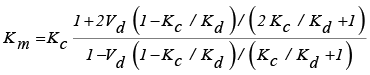(1)

where Vd is the volume fraction of dispersed phase.

Yingguang Liu et al., concluded that the thermal conductivity of nano-crystalline materials dependent on grain-size, and it decreases with the decreasing of grain size. This can be explained that with the decreasing of the grain size, boundary scattering effect is more and more significant.

So, Madan Singh et al., they found a mathematical relationship between thermal conductivity and nano grain size as follows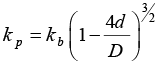(2)

In which kp thermal conductivity of nanomaterial, kb is thermal conductivity of bulk material, d is the diameter of the nanosolid and D is the diameter of the spherical nanosolids.

The thermal conductivity k of an insulating solid can be calculated from the kinetic theory of phonons in solids and expressed as: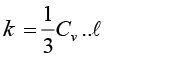(3)

Where Cv: is the heat capacity per unit volume, v: is the average phonon velocity and l: is the mean free path of a phonon.

Assume that heat capacity (Cv) in porous materials depends on porosity P as:

Cv = Cvo(1 − P) (4)

where Cvo is the heat capacity at P = 0.

The phonon mean path in porous materials must primarily be estimated by considering various phonon scattering processes. We here use a simple model to estimate it. We consider that the phonon mean free path l in a nano-porous material is determined by two scattering processes, scattering by pores and by others.

Thermal diffusivity is a material parameter describing the movement of the isothermal surface during the heat flow through the material. Thermal diffusivity Dth characterizes a material in a complex way, because it includes the heat conductivity (K), specific heat (C) and the mass density (ρ) of the material: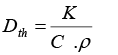(5)

The value of the thermal diffusivity quantity depends on the chemical composition of the material and its internal structure. Investigations of measurement techniques for thermo-physical properties of ceramic materials are of great importance in relation to the development of new and advanced materials. .

The general increase in volume with temperature is mainly calculated by the increased amplitude of atomic vibrations about a mean position. As the lattice energy increases, the increased amplitude of vibration between equivalent energy positions leads to a high value for the atomic separation corresponding to a lattice expansion .

The change in length with temperature for a solid material may be expressed as follows: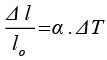(6)

Where Δl is the change in the length, lo is the original length, á coefficient of linear thermal expansion (CTE) and ΔT defined as the change in temperature. 

The coefficient of thermal expansion increases rapidly at low temperature and reaches a nearly constant value above the Debye characteristic temperature èD. Normally there is a continued increase observed above this temperature, resulting from the formation of Frenkel or Shottky defects. 

MATERIALS AND SPECIMENS PREPARATION

Yttria-Partially Stabilized Zirconia (Y-PSZ) was prepared by adding 8 wt% of yttria to zirconia and the spinel: Al2O3 (71.67 wt%) and MgO (28.33 wt%). As well as, different weight percentage of MgO-Al2O3 (5,10,15,20 and 25 wt%) was added to the Y-PSZ mixture. After mixed the composition with 10 wt% of PVA as a binder, the specimens was pressed using hydraulic press at pressure 300 MPa for 30 s to prepared discs with different diameters and thicknesses.

The sintering process was obtained by using an electrical programmable furnace type (NABERTHERM-P310-GERMANY). 3.2, with temperature rising rate 15 oC/min up to 1550 oC at sintering soaking time 4 hr to complete the Process of grain growth and get the required phase.

All materials were supplied by the companies listed in the Table 1.

Test Methods

Grain size was calculated by using Atomic Force Microscopy (AFM) type SPM-AA 3000 Angstrom (USA).

The thermal conductivity measurements (K in W/m k) are carried out by using Lee’s method . Assuming, that the heat flow in the specimen is the mean of the quantities of heat flowing into it and out of it, therefore: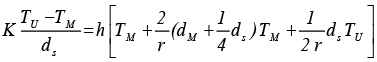(7)

where, TU: temperature of disk (U), TM: temperature of disk (M), r: radius of the disk, dM: thickness of the disk M, ds: thickness of the disk (S) (specimen) and h: heat loss per second/cm2 for one degree in excess of temperature of disk over that of the enclosure, determined from following equation: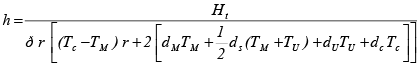(8)

In which dc and Tc: thickness and temperature of disk(c) respectively.

Lee’s disk system is isolated from external effects by using glass desiccator’s. When the system is linked to an electric power supply; then the power in watt is:

Ht = I V (9)

where V: is The Voltage (6 V) and (I) is the current equal to 0.25 A.

The Mean Specific Heat Capacity of materials was measured in accordance standard test method (ASTM C351 and E1269) by using “Calorimeter”. The following equation using for mean specific heat capacity calculation: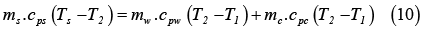where: ms: mass of specimen, cps: mean specific heat capacity for specimen, Ts: temperature of heated specimen (100°C) before putting inside the calorimeter, T2: final temperature after putting the heated specimen inside Calorimeter, T1: initial temperature of water (0°C), mw: mass of water, cpw :
specific heat capacity of water (4200 J. kg-1.K-1), mc: mass of calorimeter, cpc : specific heat capacity of calorimeter.

Hence, the thermal diffusivity can be calculated by the equation 5.

Push rod dilatometers are used to measure the thermal expansion of the specimens According to specifications (ASTM C372). The specimen is placed in a holder with a rod pushing against one end of the specimen.

As the specimen is heated, the change in length of the specimen is measured by a dial gauge attached to the opposite end of the push rod.

The linear expansion measuring system is calibrated on standard specimens (alumina, mullite, and silicon carbide) and the error present is calculated from background expansion, caused by the expansion of stage furnace and the rod expansion.

The thermal expansion percentage is calculated from the equation: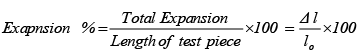(11)

RESULTS AND DISCUSSION

The topography images of sintered specimens taken by using AMF were illustrated in the Fig. 1, the measurement range for all specimens is (10×10 µm). This Figure shows 3D topographies profiles under different MAS additives, where the max high (peak-peak) of the grain size was decreased with spinel additions increases. The value has become 51.37 nm for sintered specimens of Y-PSZ with 5 wt% spinel and listed in Fig. 1a. As well as, the max high of the grain size was gradually decreasing to 25.78 nm, 18.5 nm, 5.15 nm and 3.62 nm with 10, 15, 20 and 25 wt% of spinel, respectively, as shown in Fig. 1(b, c, d and e).

The grain size was decreased with nano spinel additions increases with range from 624 nm to 109 nm and the porosity was low and less than 5 %, where reaching a minimum value of 2.5 % when adding 25 % MgO.Al2O3.

Table 2 shows the effect of MAS additions on thermal conductivity (K), where, K was reduced significantly with percentage additions of spinel. Especially for 5 % and 10% added ratios. As for additions 15, 20 and 25% of spinel, the values of K are convergent. Generally, thermal conductivity values were ranged from 5.5 to 5.64 W/m.K, i.e, the percentage of decrease between the highest and lowest values is 2.5 %. There are three important factors affecting the behavior of thermal conductivity. The first is the thermal conductivity values of starting materials for sintered specimens components, where the K value of MAS is higher than K of Y-PSZ. Therefore this factor is supposed to increase the conductivity but the effect of other factors was greater. Thus, the effect of materials characteristics was clear at range more than 10 wt% spinel. The second factor is porosity, which has a significant and clear effect especially at high percentage . In spite of the reverse relationship between porosity and thermal conductivity , the porosity did not decrease significantly because the changes are not large.

The third most important factor is grain size. Fig. 2 shown the relation between thermal conductivity and grain size. It can be seen that there is a substantial reduction in thermal conductivity as the grain size decreases. The variation of thermal conductivity values was explained by the dispersion of grain size in specimens resulting in different strengths of phonon scattering on the grains.

The large dispersion of grain size in nanostructured materials and the inevitable appearance of porosity should lead to the enhancement of phonon scattering and a reduction of thermal conductivity .

The specific heat capacity is an assessable physical quantity that illustrates the capacity of a body to store heat when the sample temperature is altered .

The same three factors (material characteristics, porosity and grain size), which have been discussed in thermal conductivity will affect specific heat capacity. It has known that heat capacity of Y-ZrO2 is lower than MAS . This factor played a major role to increasing specific heat capacity (Cp) with spinel additions increases. Table 2 are listed the values of specific heat capacity, where the Cp was increased by 10% when the additions of spinel increased from 5 wt% to 25 wt%, while the overall percentage of the increase was 27 % at additives from 5 wt% to 20 wt%. the high value of Cp is 615 J/kg.K which means that it takes about 615 J of energy to raise the temperature of 1 kg of material by 1 K. the relationship between porosity and Cp is reverse, , so the decreasing of porosity with increased the amount of spinel additions has contributed to increased Cp.

From Fig. 3 does not necessarily mean that the Cp was decreased with grain size, because that is difficult to explain and no functional relationship between them. Therefore, the decreasing behavior was came from obvious deference among the Cp values of materials.

However, S. Bottiglieri  found a relation between grain size and Cp, where an increase in thermal conductivity causes an increase in predicted grain size where an increase in specific heat capacity will cause a decrease.

Thermal diffusivity (Dth) is a thermo physical parameters unique for every materials which is a measure of the heat flux rate through a medium and depends on the structure and composition of the ceramic material. Physically, thermal diffusivity expresses how fast heat propagates across a material, being an important variable in transient heat transfer conditions.

The Dth values as a function of spinel additions for sintered specimens are listed in Table 2. Generally, thermal diffusivity values was decreased with spinel addition increases from 2.47 to 1.53 mm2/s. This decreasing is due to the composition and grain size, in spite of the effect of the reverse relationship between porosity and diffusivity , so the effect of porosity is little.

Grain size dependence on thermal diffusivity and plotted as shown in Fig. 4 to observe the effect of grain size on thermal diffusivity. It was found that thermal diffusivity increased with grain size increases, due to the decreasing contribution of grain boundaries to phonon scattering when the grain size increased from nano to micro sized grains. For specimens that containing smaller grains and hence more grain boundaries, phonon-grain boundaries scattering is much stronger .

B. Raneesh et al.  was concluded that thermal diffusivity increasing with grain size increases and Dth shows a gradual increase with grain size.

The percentage of thermal expansion (ΔL/Lo) was increased linearly with temperature increases, as shown in Fig. 5. Linear proportion does not continue up to 1000 oC, so that the expansion reaches a state of semi-stability at temperature below 1100 oC. Semi-stabile of thermal expansion is more distinguished when spinel addition increases.

Thermal expansion of ceramics due to the negligible small probability of electronic excitation over the gap, there is nothing left in a rigid lattice insulator that could account for the pronounced thermal expansion typically observed in experiments. These expansion are, on the other hand, key to many engineering applications of materials sciences (different pieces simple have to fit together over varying temperatures). Likewise, for structural phase transitions like monoclinic to tetragonal for ZrO2 upon heating and finally melting the static lattice approximation fails with a vengeance [22,23]. Thermal expansion is a very important parametric quantity for interpreting the thermodynamic and thermoelastic behavior of solids at high temperature. Thermal expansion dependence of the temperature up to 1000 oC for isobars of specimens with quasiharmonic Deby model calculations are presented in Fig. 5. It is clearly seen that the thermal expansion increases with temperature, and the effects of temperature become less and less pronounced resulting in linear high-temperature behavior.

Ore results show the thermal expansion converges to a constant value at high temperature and it is called the Deby temperature θD, where at this temperature the normal mode of vibration of crystal’s become highest values, i.e., the highest temperature that can be achieved due to a single normal vibration .

The slope of the linear behavior as shown in Fig. 5, represents the values of thermal expansion coefficient (TEC) and this values of (TEC) are listed in Table 2. The TCE was decreased with MAS additions increases and the values of TEC for spinel, porosity and grain size were major factors affecting on TEC of Y-PSZ-MAS composites. The CTE of MAS is 7.6×10-6 (oC)-1  and 10.4×10-6 (oC)-1 for Y-PSZ . this difference leading to decreasing TEC with spinel addition increases. The TCE relates intimately to the porosity through a power law:  , where ád is TEC of porous materials, ás is TEC of solid skeleton and ϕ is the present of porosity . In spite of the reverse relationship between porosity and TEC, however, porosity did not affect significantly, due to lowering the amount of porosity in the sintering specimens.

The most important factor affective the changing of TEC is grain size. Fig. 6 shows the TEC increased with grain size increases. The increase of the apparent TEC with grain size is in particular evident for it above 100 nm. This results contradicts the behavior, so, reported in the literature about the decrease of thermal expansion with grain size. However, it should be noted the sintered ceramics with grain size exceeding 100 nm were rarely investigated. An additional feature of the coarse grained ceramics is the elongation of the specimen after thermal cycle in comparison to the original size .

CONCLUSIONS

The thermal diffusivity increased with increasing grain size due to decreased amount of grain boundaries. Nano-sized grains would lead to more phonon-boundaries scattering. The convergence of the thermal expansion coefficient of the three oxides (ZrO2, Al2O3, MgO) was very useful in reducing the microscopic cracks created during the sintering process and thus increasing the mechanical properties. Increasing heat capacity contrast decreasing thermal conductivity and thermal diffusivity with spinel additions is getting good thermal insulation products and can be used in heat energy storage.

CONFLICT OF INTEREST

The authors declare that there is no conflict of interests regarding the publication of this manuscript.